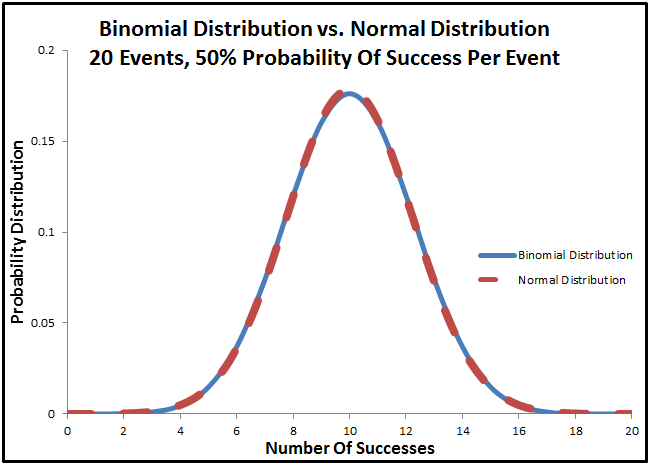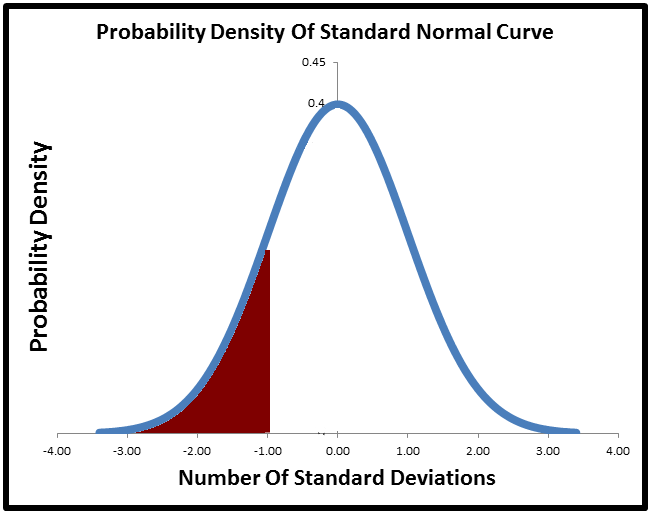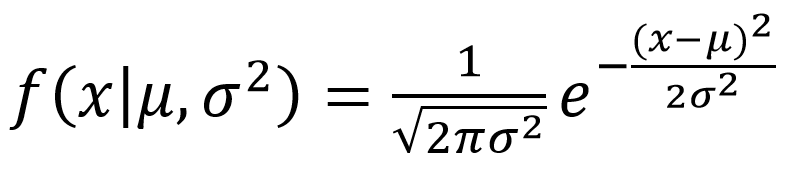# Normal Distribution Summary

This post gives the most important points to understand for the normal distribution.  If you want to see the rest my content for statistics, please go to this table of contents

Normal Curve Basic Information

• The standard normal curve is a probability distribution, which means the total area under the curve is 1.0• Like all probability density functions (PDF), it can be done as a cumulative function, i.e. sum as you go, to show a cumulative density function (CDF)• You more frequently see the normal curve plotted as a probability density function (i.e. the bell curve). But most of the time when you actually use it, such as to look up the probability of something being more than 2 standard deviations away from the mean by using a Z table, you are actually using the cumulative density function.
• To put it a different way, we more frequently care about the area under some section of the curve (the CDF at one location minus the CDF at another location) than the actual probability of any specific point on the curve.

The Real Life Meaning Of The Normal Curve

• A normal curve has a physical meaning in real life
• If you take multiple measurements of different samples, some results will end up high, some will end up low, and most will fall in the middle. The shape that results will likely be the normal curve
• The classic example of this is if you measure the height of a group of plants, i.e. how tall are different stalks of corn
• The normal curve also has an easy to duplicate mathematical meaning
• If you take a large number of independent trials of an event with 50% probability, the resulting shape will be a normal curve
• I.e. if I flip a coin 20 times and count the number of heads, and I repeat that test many times, the resulting histogram will be similar to a normal distribution
• The chart below shows the binomial distribution of 20 trials with a 50% likelihood repeated, vs the normal distribution using the same mean and standard deviation.• The more times I do that test to more similar the binomial distribution will become to the normal distribution
• This is the same reason the normal distribution exists in real life
• For instance, if we assume that a plant’s height is determined by 20 genes, and the genes the plant receives could be either tall genes or short genes, then the number of tall genes the plant gets is basically equivalent to saying how many heads will you get in 20 flips. It will follow the same distributions, which approaches the normal curve as the number of trials increases.

Using A Normal Distribution

• The normal distribution uses standard deviations as a way of fitting itself to any set of data. The standard normal distribution assumes that the standard deviation of the data set is 1.0.  You can either stretch / shrink the standard normal distribution to match your data, by multiplying it by the standard deviation, or you can shrink / stretch your data to match the standard normal distribution by dividing by the standard deviation.
• What we usually care about with a normal distribution is area under the curve up until a certain number of standard deviations
• For instance, if we want to know what percentage of the total area fall between -2 to +2 standard deviations, we would take this areaAnd we would see that 95.4% of the data falls within 2 standard deviations of the mean

• If we want to know what percentage was to the left of -1 standard deviations we would take this areaAnd see that 15.9% of the data is greater than 1 standard deviation to the left of the mean.

• Frequently this is done using a normal table, also known as a Z-table
• There are lots of good references on how to use a Z table, including this youtube video.

Level 2 Information – I recommend you get familiar with the basics of some of the other statistics topics before coming back and revisiting this in greater depth.

• Recall that the normal distribution can be generated as a series of binary events done an infinite number of times. The math of understanding binary events is the binomial distribution.  One way to approximate the binomial distribution is a normal curve. This is because the binomial distribution is just the normal curve with a finite number of events instead of an infinite number of events.
• A rule of thumb for when the normal curve is a good approximation for the binomial distribution is when the minimum of either the odds of success or failure (either p or (1-p) ) in a single trial are greater than 10. e. if I have a 50% chance of success and do 10 trials, then 0.5 * 20 = 10, so this would be a good approximation
• If I had an 80% chance of success in a single trial, and do 12 trials then I take the minimum of the odds of success (80%) or failure (20%). So this would be .2 * 12 = 2.4, so the normal curve would not be a good approximation of this binomial distribution.• The normal curve is a key component in testing for statistical significance, also known as hypothesis testing

Level 3 Information

• Not everything is a normal distribution. Some real-life events have infrequent outcomes that are more likely to occur than a normal distribution would predict.  One example of this is the financial  Occasional crashes happen with more severity than would be predicted by a normal distribution
• There are different ways of measuring a probability distribution to see how similar it is to the normal distribution
• Kurtosis is a measure of how much probability is at the center of the distribution vs at the tails
• A normal distribution has a kurtosis of 3.0, something with a fat tail would have a kurtosis greater than 3.0. This cross validated answer has a good visualization of kurtosis
• Skew is a way of measuring if the distribution is centered or not. A normal distribution is exactly symmetric.  However, a probability distribution can also be skewed left or skewed right.
• Skewed left means that the left tail is a lot longer than the right tail. This is a skewed left distribution• Skewed right (also known as positive skew, because there is more area to the right of the mean) means that the right tail has a lot more area than the left tail. This is a skewed right distribution• The normal distribution also has an equation, which is• Where µ is the mean of the data
• And σ is the standard deviation
• This is the probability distribution of the standard normal curve. Plugging in a mean, a standard deviation, and a value that you are looking for in this equation is the equivalent of using the NORM.DIST() function in Excel

Next Topic

You may want to look at Hypothesis Testing next, which heavily uses the normal distribution